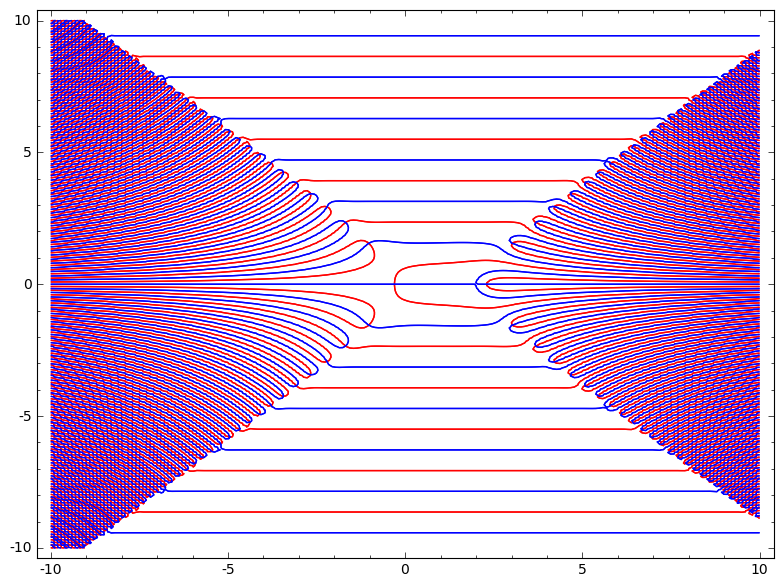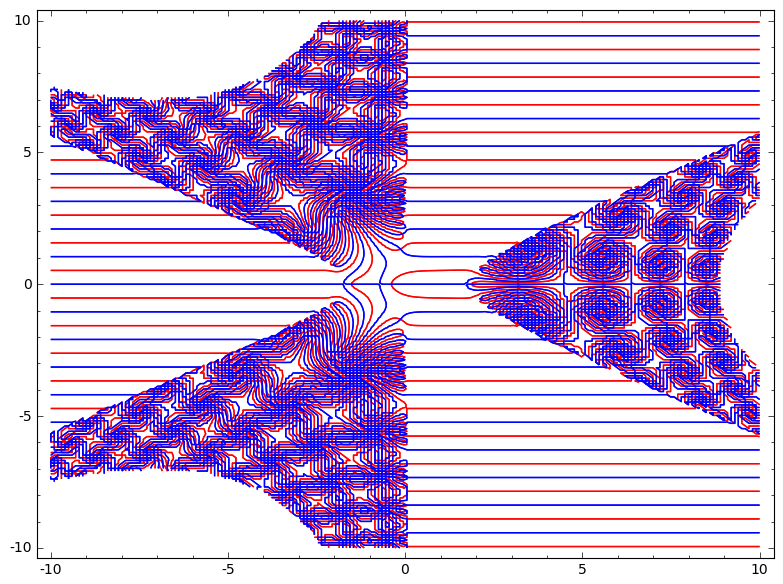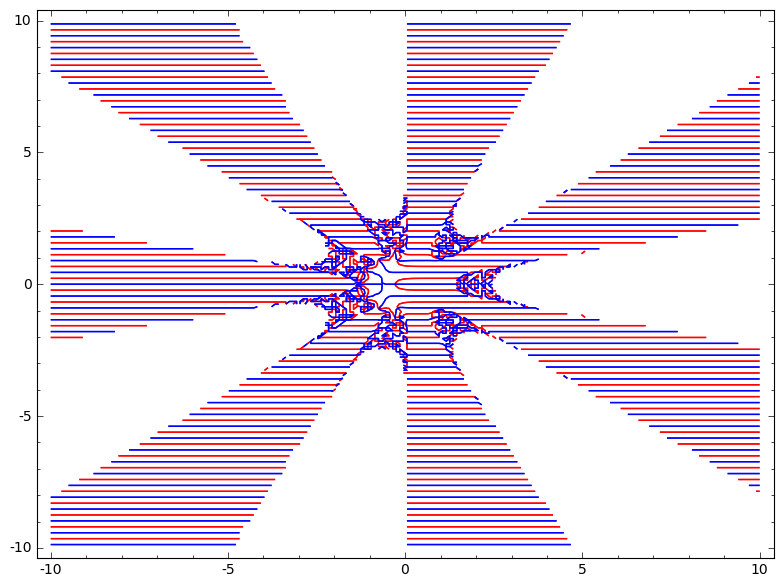## Mon Jun 24 16:28:47 EEST 2013

### XRay of \$k e^{x k} = e^{x^k}\$

XRay of \$F(x) = k e^{x k} - e^{x^k}\$. Red is \Re F(x)=0, blue is \Im F(x) = 0. Intersection of read and blue is a complex zero (modulo bugs). Counterintuitively to dumb me the number of "areas" equals \$k\$ for integer \$k\$.
k=2k=3k=7k=3, zoomPosted by j | Permanent link